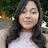# Calculating Trade Discount Rate when List Price and Net Price are Known

The trade discount rate can be calculated by using the now-familiar percentage formula Rate = Portion ÷ Base.

For this application, the amount of the trade discount is the portion, or numerator, and the list price is the base, or denominator.

## 2 thoughts on “Calculating Trade Discount Rate when List Price and Net Price are Known”

1.kyutie hoon says:

Hello! What if the List price is 80% and NIP is 7900? How will I get the list price, discount, dr, and Nip rate?

2.lety Vargas says:

Thank you